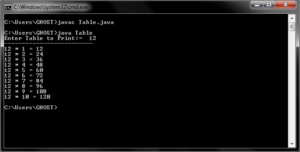Tables Printing in Java Programs

Tables are Mathematical listed down numbers which showing the results with calculated series Rows and Column.
Num * 1 = SNum
upto
Num * 10 = SNum or Num * 20 = SNum

example: 2*5=10 which means 2 number multiple by 5 which is equals to 10 and if 2 number is added 5 times its also calculated 10.(Visited 41 times, 1 visits today)

Written by:

### One thought on “Tables in JAVA”

• 02/08/2018 at 5:55 am